Test: Perimeter and Area- 2

# Test: Perimeter and Area- 2

Test Description

## 10 Questions MCQ Test Mathematics for Class 4 | Test: Perimeter and Area- 2

Test: Perimeter and Area- 2 for Class 4 2022 is part of Mathematics for Class 4 preparation. The Test: Perimeter and Area- 2 questions and answers have been prepared according to the Class 4 exam syllabus.The Test: Perimeter and Area- 2 MCQs are made for Class 4 2022 Exam. Find important definitions, questions, notes, meanings, examples, exercises, MCQs and online tests for Test: Perimeter and Area- 2 below.
Solutions of Test: Perimeter and Area- 2 questions in English are available as part of our Mathematics for Class 4 for Class 4 & Test: Perimeter and Area- 2 solutions in Hindi for Mathematics for Class 4 course. Download more important topics, notes, lectures and mock test series for Class 4 Exam by signing up for free. Attempt Test: Perimeter and Area- 2 | 10 questions in 15 minutes | Mock test for Class 4 preparation | Free important questions MCQ to study Mathematics for Class 4 for Class 4 Exam | Download free PDF with solutions
 1 Crore+ students have signed up on EduRev. Have you?
Test: Perimeter and Area- 2 - Question 1

### Direction: Six students of Class 4 are playing a Maths puzzle game. Each of the student gives a puzzle to other five numbers. Solve all the puzzles as given below: Bablu's puzzle says, "My rectangle has an area of 8 square metre and perimeter of 12 metre." What is the figure of Bablu's rectangle?

Detailed Solution for Test: Perimeter and Area- 2 - Question 1

Bablu's puzzle: Area = 8 square metres Perimeter = 12 metres.

Figure: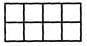Therefore, the answer is option (d).

Test: Perimeter and Area- 2 - Question 2

### A customer asked for two extra-large bed sheets. What is the perimeter of each bed sheet? length of bedsheet= 5m breadth= 4m

Detailed Solution for Test: Perimeter and Area- 2 - Question 2

Perimeter of extra-large bed sheet = 5m + 5m + 4m + 4m = 18m.

Therefore, the answer is option (b) 18m.

Test: Perimeter and Area- 2 - Question 3

### A customer asked for 2 small bed sheets, 1 large bed sheet and 2 deluxe bed sheets. How much did he/she have to pay? Price of small bed sheet = Rs.100  Price of deluxe bed sheet = Rs.500 Price of large bed sheet = Rs.300

Detailed Solution for Test: Perimeter and Area- 2 - Question 3

Price of 2 small bed sheets = Rs.100 + Rs.100/- = Rs.200/-

Price of 1 large bed sheet = Rs.300/-

Price of 2 deluxe bed sheet = Rs.500/- + Rs.500/- = Rs.1000/-

Total amount the customer has to pay = Rs.200/- + Rs.300/- + Rs.1000/- =Rs.1500/-

Test: Perimeter and Area- 2 - Question 4

Direction: Read the passage given below and answer the questions that follow: Ramu Kaka is a farmer. He has a land of length 100m and width 90m. He grows vegetables in his land and sell them in the market. But due to hot weather and no rain he suffered huge loss in his vegetable selling as he could not grow vegetables in his land so he had to sell a portion of his land of area 4000 square metre. After selling his land he again sold another portion of area 2000 square metre. Then all of a sudden the weather came in the favour of Ramu Kaka and it started raining. Ramu Kaka started making profit in his vegetable selling. He then bought a portion of land of area 3000 square metre. Then after few months he again bought another portion of land of 5000 square metre. Now he has a huge land on which he grows his vegetables. Ramu Kaka is now happy and making more and more profits every day. What is the area of the land Ramu Kaka had initially?

Detailed Solution for Test: Perimeter and Area- 2 - Question 4

The area of land Ramu Kaka had initially = 100m90m = 9000 square metre

Therefore, the answer is option (c) 9000 square metre.

Test: Perimeter and Area- 2 - Question 5

Direction: Read the passage given below and answer the questions that follow: Ramu Kaka is a farmer. He has a land of length 100m and width 90m. He grows vegetables in his land and sell them in the market. But due to hot weather and no rain he suffered huge loss in his vegetable selling as he could not grow vegetables in his land so he had to sell a portion of his land of area 4000 square metre. After selling his land he again sold another portion of area 2000 square metre. Then all of a sudden the weather came in the favour of Ramu Kaka and it started raining. Ramu Kaka started making profit in his vegetable selling. He then bought a portion of land of area 3000 square metre. Then after few months he again bought another portion of land of 5000 square metre. Now he has a huge land on which he grows his vegetables. Ramu Kaka is now happy and making more and more profits every day. What area of land he owned now?

Detailed Solution for Test: Perimeter and Area- 2 - Question 5

Area of land he owned now = 6000 square metre + 5000 square metre = 11000 square metre.

Therefore, the answer is option (d) 11000 square metre.

Test: Perimeter and Area- 2 - Question 6

Find the area of the figure given below: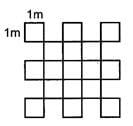Detailed Solution for Test: Perimeter and Area- 2 - Question 6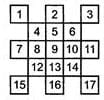Area of the given figure = 17 square metre

Therefore, the answer is option (b) 17 square meter.

Test: Perimeter and Area- 2 - Question 7

State true/false for the following statements. A: If a small square of side 1 cm is cut off from the corner, then the boundary will remain the same. B: If a small square of side 1 cm is cut off from the corner then the boundary will get increased. C: If a small square of side 1 cm is cut off the corner then the boundary will get decreased. D: All statements are false.

Detailed Solution for Test: Perimeter and Area- 2 - Question 7

Boundary of the square = 4cm + 4cm + 4cm + 4cm = 16cm. If a small square of side 1cm is cut off from its corner from the shape would become: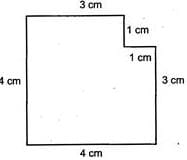Boundary of new shape = 4cm + 3cm + 1cm + 1cm + 3cm + 4cm = 16cm

Boundary remains same.

So, statement A is true and B, C, D are false.

Therefore, the answer is option  TFFF.

Test: Perimeter and Area- 2 - Question 8

Which of these is expressed in units of length?

Detailed Solution for Test: Perimeter and Area- 2 - Question 8

Perimeter is expressed in units of length.

Test: Perimeter and Area- 2 - Question 9

If area : 14 square cm : : Perimeter : ?

Detailed Solution for Test: Perimeter and Area- 2 - Question 9

Perimeter of the figure = 18cm. Therefore, the answer is option (d) 18cm.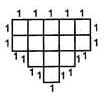Test: Perimeter and Area- 2 - Question 10

What is the perimeter of a square field whose side is 4 m?

Detailed Solution for Test: Perimeter and Area- 2 - Question 10

The side of the square field = 4 m

∴ Its perimeter =4 × 4m=16m

## Mathematics for Class 4

17 videos|68 docs|32 tests
 Use Code STAYHOME200 and get INR 200 additional OFF Use Coupon Code
Information about Test: Perimeter and Area- 2 Page
In this test you can find the Exam questions for Test: Perimeter and Area- 2 solved & explained in the simplest way possible. Besides giving Questions and answers for Test: Perimeter and Area- 2, EduRev gives you an ample number of Online tests for practice

## Mathematics for Class 4

17 videos|68 docs|32 tests